﻿ Inequalities for the <i>S</i><SUP><i>th</i></SUP> Derivative of Polynomials Not Vanishing inside A Circle

Inequalities for the Sth Derivative of Polynomials Not Vanishing inside A C...

GULSHAN SINGHOPEN ACCESSPEER-REVIEWED

Inequalities for the Sth Derivative of Polynomials Not Vanishing inside A Circle

Govt. Department of Education Jammu and Kashmir, India

Abstract

Let P(z) be a polynomial of degree n having all its zeros in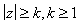, then for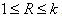, Bidkham and Dewan [J. Math. Anal. Appl. 166(1992), 191-193] proved max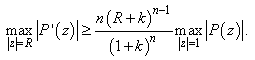In this paper, we prove an interesting generalization as well as an improvement of this result by considering the sth derivative of lacunary type of polynomials P(z) of degree n > 3.

• SINGH, GULSHAN. "Inequalities for the Sth Derivative of Polynomials Not Vanishing inside A Circle." American Journal of Mathematical Analysis 3.1 (2015): 1-4.
• SINGH, G. (2015). Inequalities for the Sth Derivative of Polynomials Not Vanishing inside A Circle. American Journal of Mathematical Analysis, 3(1), 1-4.
• SINGH, GULSHAN. "Inequalities for the Sth Derivative of Polynomials Not Vanishing inside A Circle." American Journal of Mathematical Analysis 3, no. 1 (2015): 1-4.

 Import into BibTeX Import into EndNote Import into RefMan Import into RefWorks

1. Introduction and Statement of Results

Letbe a polynomial of degree n and P’(z) its derivative, then it is known that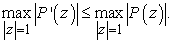(1)

The above result, which is an immediate consequence of Bernstein's inequality on the derivative of a trigonometric polynomial is best possible with equality holding for the polynomial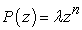, whereis a complex number.

If we restrict ourselves to the class of polynomials having all their zeros in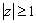, inequality (1) can be sharpened. In fact, Erdös conjectured and later Lax  proved that if P(z)≠ 0 in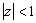, then(2)

On the other hand, if the polynomial P(z) of degree n has all its zeros in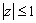, then it was proved by Turán , that max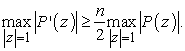(3)

Inequalities (2) and (3) are best possible and become equality for polynomials which have all zeros on.

Inequality (2) was refined by Aziz and Dawood  by showing that under the same hypothesis that(4)

Equality in (4) holds for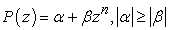.

For the class of polynomials P(z) of degree n having all their zeros in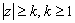, Malik  proved: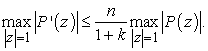(5)

Inequality (5) was further improved by Govil  who under the same hypothesis proved:(6)

Chan and Malik  obtained a generalization of (5) by considering the lacunary type of polynomials and obtained the following:

Theorem A: Let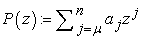,be a polynomial of degree n having all its zeros in, then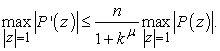(7)

The result is best possible and extremal polynomial is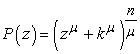; where n is a multiple of.

The next result was proved by Pukhta , who infact proved:

Theorem B: Let,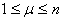be a polynomial of degree n having all its zeros in, then(8)

The result is best possible and extremal polynomial is; where n is a multiple of.

Bidkham and Dewan  obtained a generalization of (5) by proving the following result:

Theorem C: Let P(z) be a polynomial of degree n having all its zeros in, then for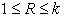(9)

The result is best possible and equality holds for P(z) = (z + k)n.

In this paper, we prove the following generalization as well as an improvement of Theorem C by considering the sth derivative of P(z).

Theorem 1: If, n>3,is a polynomial of degree n having all its zeros in, then for, and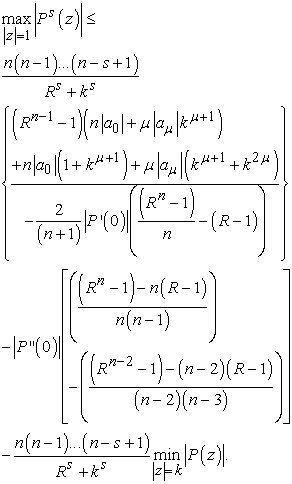2. Lemmas

For the proof of above theorem, we need the following lemmas. The first result is due to Qazi [9, Lemma 1].

Lemma 1: If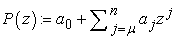,is a polynomial of degree n having all its zeros in, then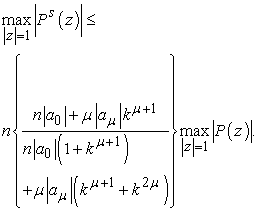The next lemma is due to Dewan, Kour and Mir .

Lemma 2: Letbe a polynomial of degree n, then for R1;(10)

and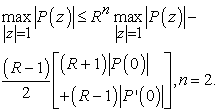(11)

Lemma 3: If, n > 3,is a polynomial of degree n having all its zeros in, then for, we have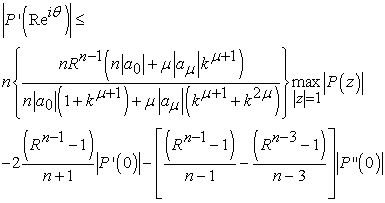Proof of Lemma 3: Since P(z) is a polynomial of degree n > 3, the polynomial P′(z) is of degree n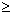3, hence on applying inequality (10) of Lemma 2 to the polynomial P′(z), we obtain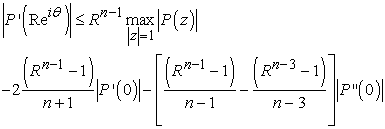This proves Lemma 3.

Lemma 4: If, n > 3,is a polynomial of degree n having all its zeros in, then for R1,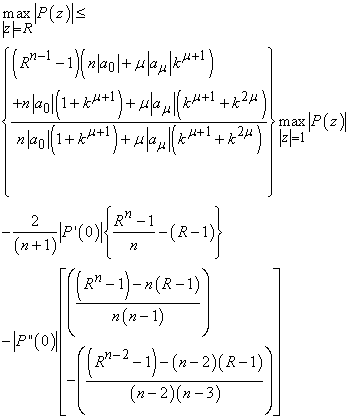Proof of Lemma 4: For each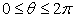and for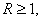we have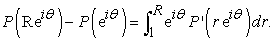Hence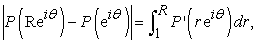(12)

which when combined with Lemma 3, gives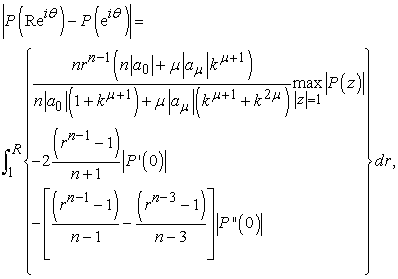which gives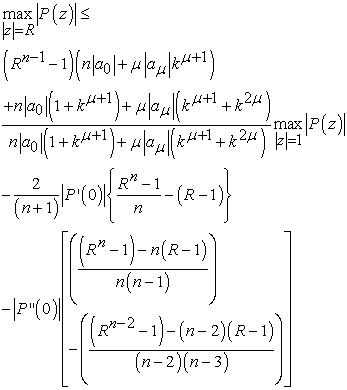Hence the proof.

Lemma 5: If P(z) is a polynomial of degree n having all its zeros in, then for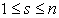.This Lemma is due to Govil .

Proof of Theorem 1: Since P(z) has all its zeros inand if, then G(z) = P(Rz) has all its zeros in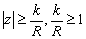, therefore by applying Lemma 5 to G(z), we obtain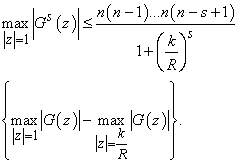which implies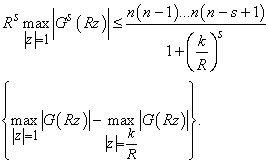which is equivalent to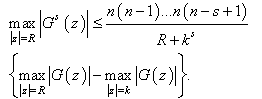(13)

Inequality (13) in conjunction with Lemma 4 yields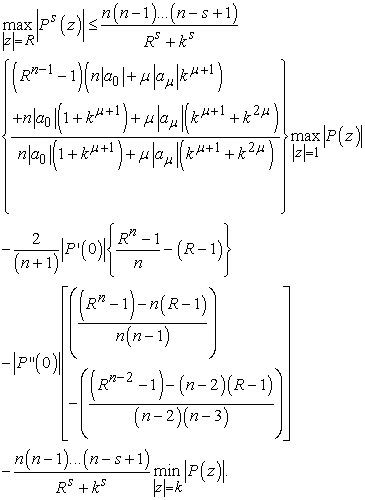The proof of Theorem 1 is completed.

References

  A. Aziz and Q.M. Dawood, Inequalities for a polynomial and its derivative, J. Approx. Theory, 54 (1988), No. 3, 306-313.In article  M. Bidkham and K. K. Dewan, Inequalities for a polynomial and its derivative, J. Math. Anal. Appl., Vol. 166 (1992), 319-324.In article  T. N. Chan and M. A. Malik, On Erdos-Lax Theorem, Proc. Indian Acad. Sci. (Math. Sci.), 92 (3) (1983), 191-193.In article  K. K. Dewan, Jagjeet Kaur and Abdullah Mir, Inequalities for the derivative of a polynomial, J. Math. Anal. Appl., 269 (2002), 489-499.In article CrossRef  N. K. Govil, Some inequalities for derivatives of polynomials, J. Approx. Theory, 66 (1) (1991), 29-35.In article  P. D. Lax, Proof of a conjecture of P. Erdos on the derivative of a polynomial, Amer. Math. Soc., Bulletin, 50 (1944), 509-513.In article  M. A. Malik, On the derivative of a polynomial, J. London Math. Soc., 2 (1) (1969), 57-60.In article  M. S. Pukhta Extremal Problems for Polynomials and on Location of Zeros of Polynomials, Ph. D Thesis, Jamia Millia Islamia, New Delhi (1995).In article  M. A. Qazi, On the maximum modulus of polynomials, Proc. Amer. Math. Soc., 115(1992), 337-343.In article CrossRef  P. Turán, Uber die Ableitung von Polynomen, Compositio Mathematica, 7 (1939), 89-95 (German).In article